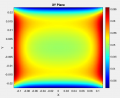# A Rectangular Helmholtz Coil and a Mathematical Puzzle

#### ZCochran98

Joined Jul 24, 2018
225
Recently, out of personal curiosity and with some time to kill, I was working on some equations for modeling the magnetic field of a rectangular Helmholtz coil (which, as it turns out, kind of sucks - see the second half of this post). In the process, however, I had to deal with the following integral (derived from the Biot-Savart Law):

$\int\frac{\mathrm{d}u}{\left(u^2+a^2\right)^{3/2}}$

This evaluates out to:

$\int\frac{\mathrm{d}u}{\left(u^2+a^2\right)^{3/2}} = \frac{1}{a^2}\frac{u}{\sqrt{u^2+a^2}} + C$

This is all fine and dandy. However, here lies the trap: you can, technically, simplify the equation. In theory, the right-hand-side could be evaluated to:

$\frac{1}{a^2}\frac{u}{\sqrt{u^2+a^2}} = \frac{1}{a^2}\frac{1}{\sqrt{1+\left(\frac{a}{u}\right)^2}}$

I'll put the answer below (I'd put it in a spoiler, but it doesn't seem that I can put equations in spoilers....), but for those of you up to a mathematical puzzle, I present you this: how did I make this simplification? And why is it wrong? What can be done to correct it?
.
.
.
.
.
.
The simplification I made is simply multiplying top and bottom by $$u^{-1}$$, which is equivalent to multiplying by 1. In theory, this should work - it's a perfectly standard trick for dealing with ugly rationals. However, there's a problem (which is what makes the simplification in its simplest form incorrect). The failure lies in the sign of $$u$$. If $$u \geq 0$$, the simplification (or the limit of the simplification as $$u \rightarrow 0$$) is valid. But when $$u < 0$$ there's a sign mismatch, thanks to the weirdness that comes with squares and roots. As an example, if $$u = -1$$, then the left-hand-side evaluates to $$-\frac{1}{a^2}\frac{1}{\sqrt{1+a^2}}$$, while the right hand side is $$\frac{1}{a^2}\frac{1}{\sqrt{1+a^2}}$$. This is, obviously, a contradiction if the "simplification" were, in fact, completely mathematically correct. To correc this issue, we just need to add the signum function (denoted here as $$\sigma(\cdot)$$) to the equation to properly write the simplification:

$\frac{1}{a^2}\frac{u}{\sqrt{u^2+a^2}} = \frac{1}{a^2}\frac{\sigma(u)}{\sqrt{1+\left(\frac{a}{u}\right)^2}}$

Whether or not this can be called a "simplification" at this point is up for debate. Computationally, I haven't sat down and evaluated which is faster for a computer to implement (it depends on how fast multiplications and divisions are compared to function calls and comparisons are).

To be honest, it took me an embarrassingly-long time (a solid three or four hours) of puzzling over why my field plots were very wrong when evaluating them in MATLAB to finally figure out that there was a problem here. But hey: that's how we learn.

And, for those of you who are curious, what I ultimately ended up deriving for the magnetic field equations for a rectangular Helmholtz coil are shown below (and, as will be seen, there's a reason nobody ever does the rectangular Helmholtz coil as an assignment when teaching electromagnetics - at least, not introductory electromagnetics). If the $$n$$-turn each coil pair, running a current of $$I$$, is a rectangle of dimensions $$w\times L$$, oriented so the width dimension runs along the y-axis and the length dimension runs along the x-axis, centered at origin, separated by a gap of $$h$$ along the z-axis with a material between them with a permeability of $$\mu = \mu_r\mu_0$$, then we can define the following equations:

$a_{\sigma_1\sigma_2}^2 \equiv \left(y-\frac{\sigma_1 w}{2}\right)^2 + \left(z - \frac{\sigma_2 h}{2}\right)^2$
$b_{\sigma_1\sigma_2}^2 \equiv \left(x-\frac{\sigma_1 L}{2}\right)^2 + \left(z - \frac{\sigma_2 h}{2}\right)^2$
$\boldsymbol{N}^x_{\sigma_1 \sigma_2} \equiv -\left(z - \frac{\sigma_2 h}{2}\right)\boldsymbol{j} + \left(y-\frac{\sigma_1 w}{2}\right)\boldsymbol{k}$
$\boldsymbol{N}^y_{\sigma_1 \sigma_2} \equiv \left(z - \frac{\sigma_2 h}{2}\right)\boldsymbol{i} + \left(x-\frac{\sigma_1 L}{2}\right)\boldsymbol{k}$
$\boldsymbol{B}^x_{\sigma_1 \sigma_2} \equiv \frac{\mu n I}{4\pi}\frac{\sigma_1}{a_{\sigma_1\sigma_2}^2}\boldsymbol{N}^x_{\sigma_1\sigma_2}\left(\frac{x-\frac{L}{2}}{\sqrt{\left(x-\frac{L}{2}\right)^2+a_{\sigma_1\sigma_2}^2}} - \frac{x+\frac{L}{2}}{\sqrt{\left(x+\frac{L}{2}\right)^2+a_{\sigma_1\sigma_2}^2}}\right)$
$\boldsymbol{B}^y_{\sigma_1 \sigma_2} \equiv \frac{\mu n I}{4\pi}\frac{\sigma_1}{b_{\sigma_1\sigma_2}^2}\boldsymbol{N}^y_{\sigma_1\sigma_2}\left(\frac{y-\frac{w}{2}}{\sqrt{\left(y-\frac{w}{2}\right)^2+b_{\sigma_1\sigma_2}^2}} - \frac{y+\frac{w}{2}}{\sqrt{\left(y+\frac{w}{2}\right)^2+b_{\sigma_1\sigma_2}^2}}\right)$
$\boldsymbol{B} = \sum_{\sigma_{1,2}=\pm 1} \left(\boldsymbol{B}^x_{\sigma_1 \sigma_2} + \boldsymbol{B}^y_{\sigma_1 \sigma_2}\right)$

I'm pretty sure I summarized those correctly, from my notes and MATLAB script....

Below is a 2D slice of the magnitude of $$\boldsymbol{B}$$ on the XY-plane centered at $$z = 0$$ (colorscale in Teslas) - so a plane parallel to the coils, centered between them (as it turns out this fields is mostly vertical, pointing down/into the screen if the current is running clockwise around the edge of the image). 15625 turns of wire each (or $$125^2$$ - roughly a 1-inch by 1-inch cross-sectional wire bundle area of each side of the coil if using 32AWG), running 4 A of current (yes, I know, practically, 32 AWG has an ampacity of 1/2 A, but this current was leftover from toying around with 20 AWG-based calculations, and should be fine, if implemented, if the current is pulsed for a very, very short amount of time. Maybe.), with a soft iron core the coils share ($$\mu_r \approx 4000$$). The coils have dimensions of $$w = 5$$ cm, $$L = 25$$ cm, and $$h = 2$$ cm.A rough, rough estimate of the amount of wire this would require is 18.75 km of 32 AWG, assuming perfect squares for each coil (so ignoring the "spiraling" extra length). That much wire would be roughly 12.3 lbs, and cost about \$171.98 from Remington Industries, based on very, very cursory research (unfortunately, if I wanted to build this, I'd probably have to get a custom order, as the allowed spool sizes jump from 10 lbs to 80 lbs). It would also have a resistance of 10.1 k$$\Omega$$, roughly (so I'd need a 41 kV supply with a power rating exceeding 161.6 kW to get the desired current!). And if I did my math right, it'd, very, very roughly, have an inductance of 1.7 H (could be wrong on that - calculating flux of this thing is a pain). I don't even want to think about the parasitic capacitances....

All that work, for about 50 mT (500 G) of magnetic field strength. Hoofta. It'd be simpler and cheaper, probably, to just buy a bunch of permanent, rare-earth magnets to get the same field strength, considering >10000 G (>1 T) neodymium magnets exist....

Apologies for the rambling post, but maybe some of you may find it interesting.

Tl;dr: Helmholtz coils suck to calculate their fields, and mathematical tricks are tricky sometimes....